Next: The Magnus force Up: Integration of ODEs Previous: The physics of baseball

## Air drag

A baseball in flight is subject to three distinct forces. The first is gravity, which causes the ball to accelerate vertically downwards at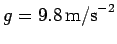. The second is air drag, which impedes the ball's motion through the air. The third is the Magnus force, which permits the ball to curve laterally. Let us discuss the latter two forces in more detail.

As is well-known, the drag force acting on an object which moves very slowly through a viscous fluid is directly proportional to the speed of that object with respect to the fluid. For example, a sphere of radius, moving with speedthrough a fluid whose coefficient of viscosity is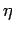, experiences a drag force given by Stokes' law:19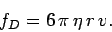(37)

As students who have attempted to reproduce Millikan's oil drop experiment will recall, this force is the dominant drag force acting on a microscopic oil drop falling through air. However, for most macroscopic projectiles moving through air, the above force is dwarfed by a second drag force which is proportional to.

The origin of this second force is fairly easy to understand. At velocities sufficiently low for Stokes' law to be valid, air is able to flow smoothly around a passing projectile. However, at higher velocities, the projectile's motion is too rapid for this to occur. Instead, the projectile effectively knocks the air out of its way. The total mass of air which the projectile comes into contact with per second is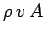, where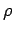is the air density,the projectile speed, andthe projectile's cross-sectional area. Suppose, as seems reasonable, that the projectile imparts to this air mass a speed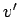which is directly proportional to. The rate of momentum gain of the air, which is equal to the drag force acting on the projectile, is approximately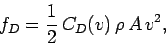(38)

where the drag coefficient,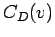, is a dimensionless quantity.

The drag force acting on a projectile, passing through air, always points in the opposite direction to the projectile's instantaneous direction of motion. Thus, the vector drag force takes the form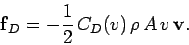(39)

When a projectile moves through air it leaves a turbulent wake. The value of the drag coefficient,, is closely related to the properties of this wake. Turbulence in fluids is conventionally characterized in terms of a dimensionless quantity known as a Reynolds number:20(40)

Here,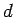is the typical length-scale (e.g., the diameter) of the projectile. For sufficiently small Reynolds numbers, the air flow immediately above the surface of the projectile remains smooth, despite the presence of the turbulent wake, andtakes an approximately constant value which depends on the projectile's shape. However, above a certain critical value of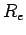--which corresponds tofor a smooth projectile--the air flow immediately above the surface of the projectile becomes turbulent, and the drag coefficient drops: i.e., a projectile slips more easily through the air when the surrounding flow is completely turbulent. In this high Reynolds number regime, the drag coefficient generally falls rapidly by a factor of between 3 and 10, asis increased, and then settles down to a new, roughly constant value. Note that the critical Reynolds number is significantly less thanfor projectiles with rough surfaces. Paradoxically, a rough projectile generally experiences less air drag, at high velocities, than a smooth one. The typical dependence of the drag coefficient on the Reynolds number is illustrated schematically in Fig. 10.Wind tunnel measurements reveal that the drag coefficient is a strong function of speed for baseballs, as illustrated in Fig. 11. At low speeds, the drag coefficient is approximately constant. However, as the speed increases,drops substantially, and is more than a factor of 2 smaller at high speeds. This behaviour is similar to that described above. The sudden drop in the drag coefficient is triggered by a transition from laminar to turbulent flow in the air layer immediately above the ball's surface. The critical speed (to be more exact, the critical Reynolds number) at which this transition occurs depends on the properties of the surface. Thus, for a completely smooth baseball the transition occurs at speeds well beyond the capabilities of the fastest pitchers. Conversely, the transition takes place at comparatively low speeds if the surface of the ball is rough. In fact, the raised stitches on the otherwise smooth regulation baseball cause the transition to occur at an intermediate speed which is easily within the range of major league pitchers. Note that the magnitude of the drag force is substantial--it actually exceeds the force due to gravity for ball speeds above about 95mph.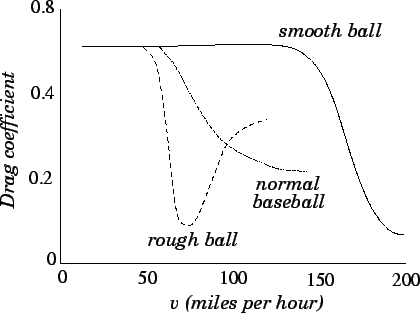The above discussion leads to a number of interesting observations. First, the raised stitches on a baseball have an important influence on its aerodynamic properties. Without them, the air drag acting on the ball at high speeds would increase substantially. Indeed, it seems unlikely that major league pitchers could throw 95mph fastballs if baseballs were completely smooth. On the other hand, a scuffed-up baseball experiences even less air drag than a regulation ball. Presumably, such a ball can be thrown faster--which explains why balls are so regularly renewed in major league games.

Giordano21 has developed the following useful formula which quantifies the drag force acting on a baseball:(41)

where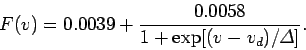(42)

Here,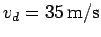and.Next: The Magnus force Up: Integration of ODEs Previous: The physics of baseball
Richard Fitzpatrick 2006-03-29